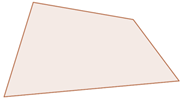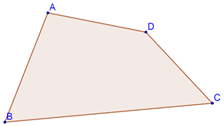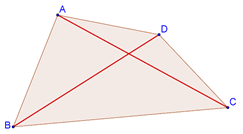# What is a Quadrilateral?

Go back to  'Triangles-Quadrilaterals'

A quadrilateral is a geometric figure formed by joining four points, any three of which are non-collinear, in some order. In other words, a quadrilateral is any closed, four-sided figure. Two examples are shown below:We note that a quadrilateral has four sides, four angles, and four vertices. When we have to specify a quadrilateral, we do it keeping in mind the order of the vertices. For example, consider the following quadrilateral:We could name this quadrilateral ABCD, BCDA, ADCB, DCBA, etc, but we cannot name it ACBD, BACD, etc., since in the latter names, the order of the vertices is wrong.

A quadrilateral ABCD will have two diagonals: AC and BD.